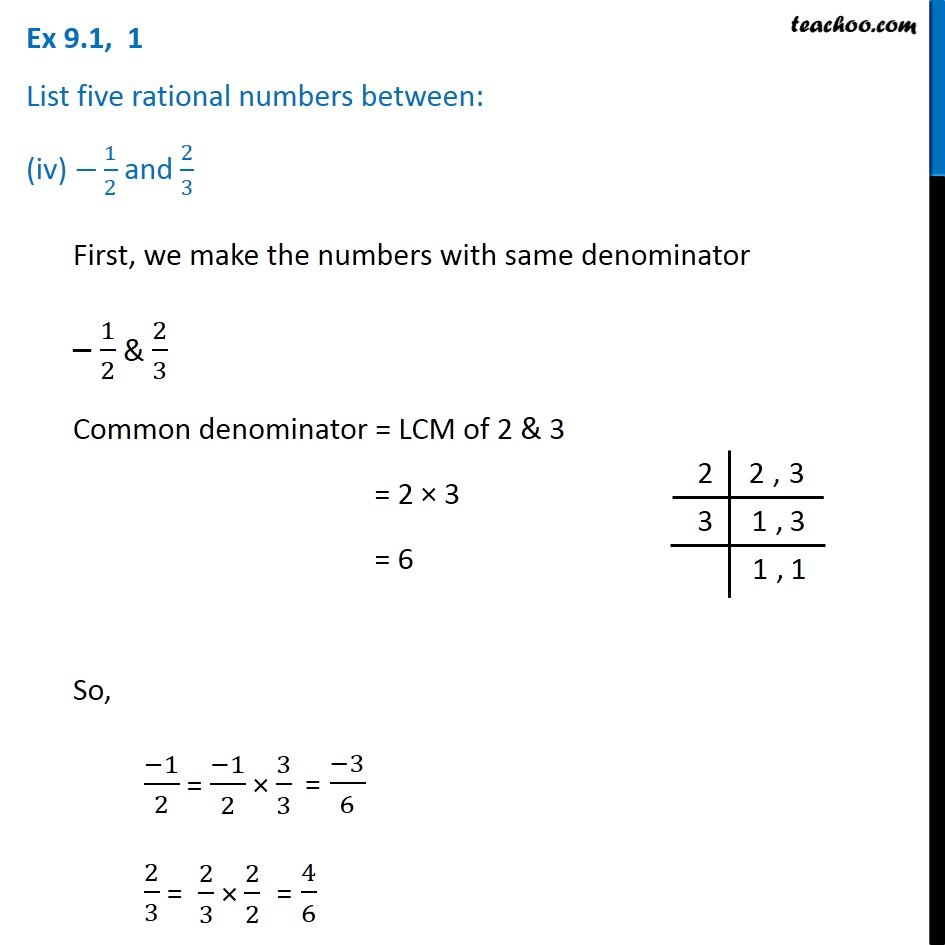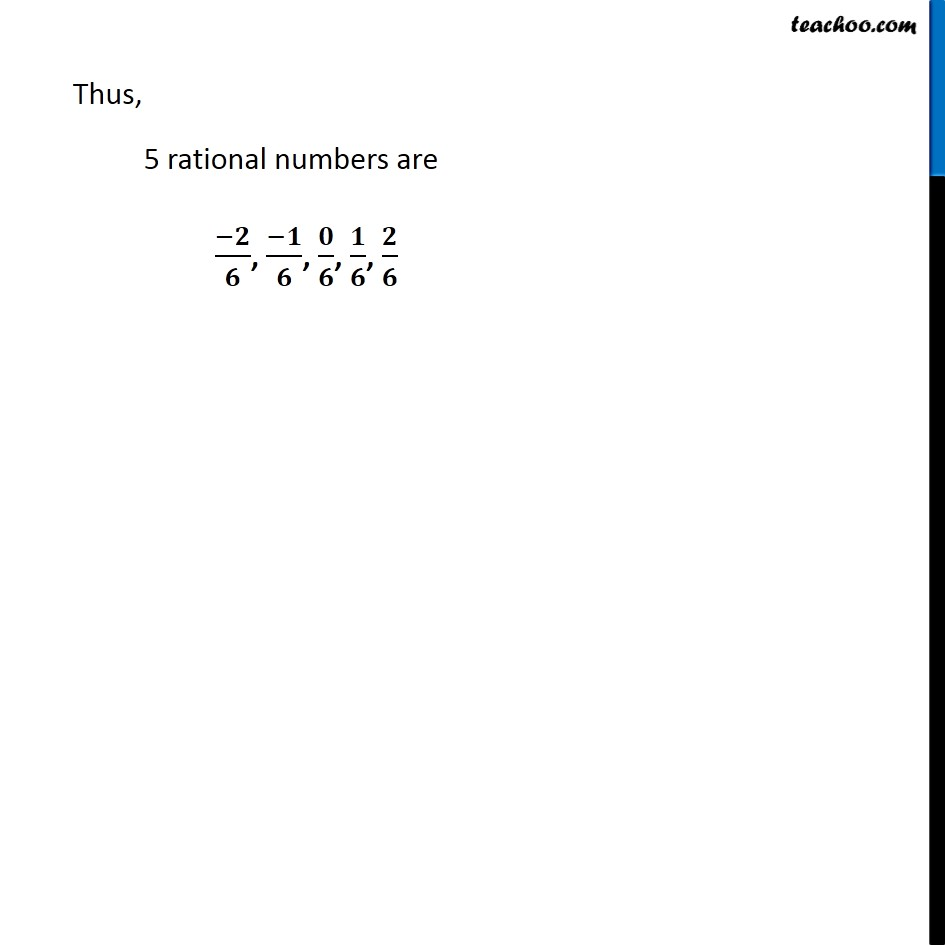1. Chapter 9 Class 7 Rational Numbers
2. Serial order wise
3. Ex 9.1

Transcript

Ex 9.1, 1 List five rational numbers between: (iv) −1/2 and 2/3 First, we make the numbers with same denominator – 1/2 & 2/3 Common denominator = LCM of 2 & 3 = 2 × 3 = 6 So, (−1)/2 =(−1)/2 × 3/3 = (−3)/6 2/3 = 2/3 × 2/2 = 4/6 Thus, 5 rational numbers are (−𝟐)/𝟔, (−𝟏)/𝟔, 𝟎/𝟔, 𝟏/𝟔, 𝟐/𝟔

Ex 9.1